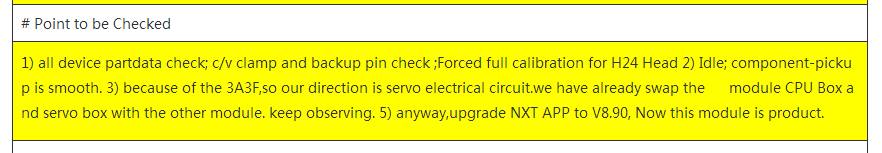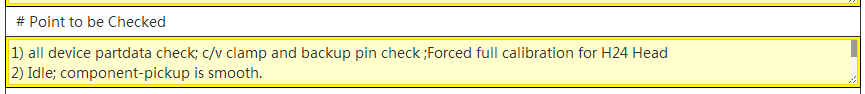NPOI 读取excel单元格内容 并写入数据表中 文本段落问题

npoi 读取excel单元格 内容写到数据库里，excel单元格内容是有换行的多行内容，写到表里后，label预览内容时无换行，但在修改状态下 textbox有换行显示正从，请问label 怎么解决？

`````` HSSFCell Point = (HSSFCell)sheet.GetRow(65).GetCell(0);
...
Point.SetCellType(CellType.STRING);
...
model.Point = Point.StringCellValue.Trim();
...
``````

``````  this.L_Point.Text = (model.Point.Replace(Char.ConvertFromUtf32(32), "&nbsp;")).Replace(Char.ConvertFromUtf32(13) + Char.ConvertFromUtf32(10), "<br />");
```````````` this.t_Point.Text = model.Point;
``````2个回答